• 网友回帖 (20)
•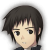Joyzhou 发表于 4个月前 没有 特殊字符的话计算Char的总和更简单 --- 共有 1 条评论 --- loyal(4个月前)：总和不适用含有差位的字母
•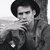LefiBelief 发表于 4个月前 略微改了一下 ```public static boolean compareCharacter(String a, String b) { if (Objects.equal(a, b)) { return true; } if (StringUtils.isEmpty(a) || StringUtils.isEmpty(b) || a.length() != b.length()) { return false; } char[] arrayA = a.toLowerCase().toCharArray(); char[] arrayB = a.toLowerCase().toCharArray(); Arrays.sort(arrayA); Arrays.sort(arrayB); return Arrays.equals(arrayA, arrayB); }``` --- 共有 1 条评论 --- fkg(4个月前)：更严谨了，学习了~
•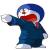loyal 发表于 4个月前 我也写了一版,里面多了个map,主要是为了记录每个字母出现的位置,用于重复字母标记而已. 开始没有写那么长,反正写着玩... ``````public static boolean difString(String a, String b) { if (a == null || b == null || a.length() != b.length()) { return false; } a = a.toLowerCase(); b = b.toLowerCase(); int len = a.length(); Map table = new IdentityHashMap<>(); for (int i = 0; i < len; i++) { char x = a.charAt(i); int m = b.indexOf(x); if (m != -1) { Map.Entry e = table.entrySet().stream().filter(me -> me.getKey().equals(x)).max((o1, o2) -> -1).orElse(null); Integer k = e==null?null:e.getValue(); if (k == null) { table.put(new Character(x), m); } else { m = b.indexOf(x, k + 1); if (m == -1) { return false; } table.put(new Character(x), m); } } else { return false; } } System.out.println(table); return true; }``````
•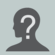freezingsky 发表于 4个月前 如果明确是字母的话, 转成ASCII的数字 , sum一下总和, 比较一下. --- 共有 1 条评论 --- loyal(4个月前)：肯定不行.那只是个和校验.遇到AD和BC你能分得出来?
•caoshijia 发表于 4个月前 应该先排序，排序完再循环逐个下标比较是否相同就行了
•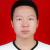learn_progress 发表于 4个月前 将字母拆成数组，再判断两数组的互相补集是否一样。
•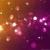Courage 发表于 4个月前 来个不一样的版本（如果明确是字母的话） ``````private static int toBitmap(String str) { int bm = 0; for (int i = 0, l = str.length(); i < l; i++) { char c = str.charAt(i); if (c >= 'a' && c <= 'z') { bm |= 1 << (c - 'a'); } else if (c >= 'A' && c <= 'Z') { bm |= 1 << (c - 'A'); } } return bm; } public static boolean compareAlpha(String a, String b) { if (null == a || null == b) { return false; } if (a == b) { return true; } // 如果字符串长度需相同 if (a.length() != b.length()) { return false; } return toBitmap(a) == toBitmap(b); }``````
•热心市民王先生 发表于 4个月前 比较每个字母出现的次数
•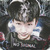吕世昊 发表于 4个月前 ```public static void main(String[] args) { int count1=0, count2=0; String a1="slqees"; String a2="sqeesl"; if(a1.length()==a2.length()){ for(int i=0;i
•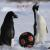绿水本无忧 发表于 4个月前 ascii排序  直接匹配不可以么  如果不可以  专程数组集合升序排  求交集